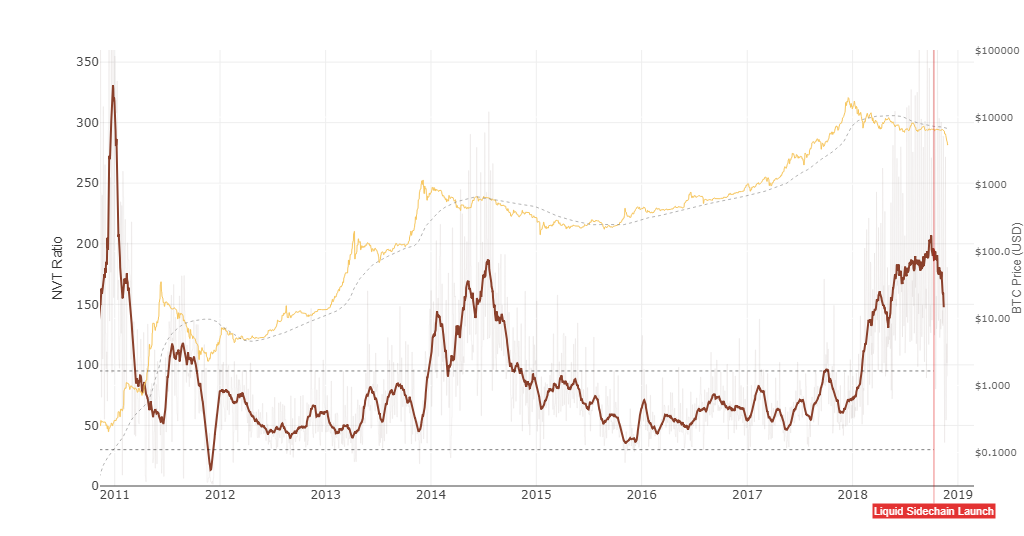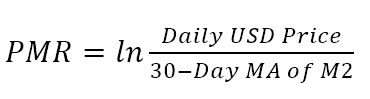# Valuations for Crypto

All you need to know about Crypto valuations;

Cryptoassets, unlike traditional assets, do not have a fixed method of valuation. Cryptoassets are a relatively new asset class which require a new analytical outlook into investing and their valuation methods. They do not share the same attributes as traditional assets so what may work for bonds or equities may not work for cryptoassets as they do not have earnings, shares or cash flows.

We use multiple valuations that differ from asset to asset. The following are some of our valuation methods; their use differs based on the nature of the crypto asset and can change over time.

## Intrinsic Valuations

Equation of Exchange is a macroeconomic model that is used to compare supply, velocity of transactions, price levels and index of expenditure. This model is useful in valuing cryptocurrencies as it uses a measure of network value and corresponds it with other variable such as speed of spending, supply, price to derive a value of the asset.

MV=PQ
M total supply in circulation of a crypto asset, commonly referred to as supply of coins for a cryptocurrency
V average frequency of which a unit is spent
P price level
Q index of real expenditure

We follow the Chris Burniske model which is M=PQ/V, for valuations where the price of the asset is not the price of the crypto asset but it is instead the price of the utility or the resource being acquired by the crypto asset network. Following this model and applying it to bitcoin, P is the combination of asset price dealt in the market and cost of provisioning it(mining). Q represents the real-output of transactions the capturable market needs in order to function and blockchain network usage will indicate Q.

PQ becomes £/(avg)price of bitcoin, mining cost per unit x units acquired = £x

This model is used for the following reasons;

• Projecting supply issuance for a crypto asset over a specific set of time.
• Forecasting velocity in the transaction volumes of a crypto asset.
• Producing discount factors to bring future utility to the present.

The following model refers to a MV=PQ valuation of 0xProject - Fortem Capital is not the owner of this model and does not claim to be.

## Relative Valuations

The following metrics and ratios are useful for comparing similar cryptoassets and modeling relative to their value in the space;

### Network Value to Transaction Ratio (NVT)

This ratio measures the value of a crypto asset’s capitalisation and compares it to daily on-chain transaction activity. Higher NVT ratios indicate that network valuations are outperforming the value transmitted on its payment network. High NVT’s can be a sign of a bubble, indicating that the capitalization of the crypto asset exceeds the network usage.### Network Value to Transacting & Trading

Useful for valuing gateway crypto assets such as Ethereum and Bitcoin, it takes into account network usage compared to trading volumes. The reason trading is used as a denominator in valuing gateway cryptos such as Ethereum and Bitcoin is because they serve as liquidity gateways into the rest of the crypto asset market. As trading of these gateway crypto increases, the NVTT for gateways crypto assets decrease over time.

### Price to Metcalfe's Law (PMR)

We follow Ken Alabi’s template and Clearblocks further analysis when applying crypto asset prices to Metcalfe’s Law. It indicates that crypto assets such as Bitcoin, Ethereum and other digital blockchain networks follow Metcalfe’s Law. PMR is used to represent the value of a network. It states that the network's impact is the square of the number of nodes in the network e.g. if a network has 10 nodes then the value is 100 (n²). Research suggests that network valuations for cryptoassets such as Bitcoin and Ethereum can be similar to the valuation for social media networks like Facebook and Wechat. It implies that as nodes/usages increase so does the value of the network.

The formula Clearblocks have used is;As case-use for these crypto assets is very little at present, PMR allows us to view the intersection of price vs network usage. The theory takes into account the comparison between crypto assets and telecommunication networks, allowing us to forecast usage increases and have a valid case study to reference back too.